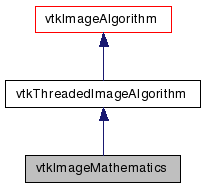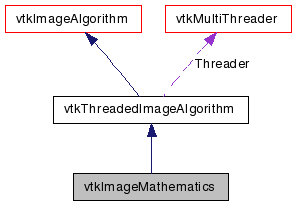# vtkImageMathematics Class Reference

`#include <vtkImageMathematics.h>`

Inheritance diagram for vtkImageMathematics:[legend]
Collaboration diagram for vtkImageMathematics:[legend]

## Detailed Description

Add, subtract, multiply, divide, invert, sin, cos, exp, log.

vtkImageMathematics implements basic mathematic operations SetOperation is used to select the filters behavior. The filter can take two or one input.

Tests:
vtkImageMathematics (Tests)

Definition at line 56 of file vtkImageMathematics.h.

## Public Member Functions

virtual const char * GetClassName ()
virtual int IsA (const char *type)
void PrintSelf (ostream &os, vtkIndent indent)
void SetOperationToSubtract ()
void SetOperationToMultiply ()
void SetOperationToDivide ()
void SetOperationToConjugate ()
void SetOperationToComplexMultiply ()
void SetOperationToInvert ()
void SetOperationToSin ()
void SetOperationToCos ()
void SetOperationToExp ()
void SetOperationToLog ()
void SetOperationToAbsoluteValue ()
void SetOperationToSquare ()
void SetOperationToSquareRoot ()
void SetOperationToMin ()
void SetOperationToMax ()
void SetOperationToATAN ()
void SetOperationToATAN2 ()
void SetOperationToMultiplyByK ()
void SetOperationToReplaceCByK ()
virtual void SetOperation (int)
virtual int GetOperation ()
virtual void SetConstantK (double)
virtual double GetConstantK ()
virtual void SetConstantC (double)
virtual double GetConstantC ()
virtual void SetDivideByZeroToC (int)
virtual int GetDivideByZeroToC ()
virtual void DivideByZeroToCOn ()
virtual void DivideByZeroToCOff ()
virtual void SetInput1 (vtkDataObject *in)
virtual void SetInput2 (vtkDataObject *in)

## Static Public Member Functions

static vtkImageMathematicsNew ()
static int IsTypeOf (const char *type)
static vtkImageMathematicsSafeDownCast (vtkObject *o)

## Protected Member Functions

vtkImageMathematics ()
~vtkImageMathematics ()
virtual int RequestInformation (vtkInformation *, vtkInformationVector **, vtkInformationVector *)
virtual void ThreadedRequestData (vtkInformation *request, vtkInformationVector **inputVector, vtkInformationVector *outputVector, vtkImageData ***inData, vtkImageData **outData, int extent, int threadId)
virtual int FillInputPortInformation (int port, vtkInformation *info)

## Protected Attributes

int Operation
double ConstantK
double ConstantC
int DivideByZeroToC

## Member Typedef Documentation

Definition at line 60 of file vtkImageMathematics.h.

## Constructor & Destructor Documentation

 vtkImageMathematics::vtkImageMathematics ( ) ` [protected]`

 vtkImageMathematics::~vtkImageMathematics ( ) ` [inline, protected]`

Definition at line 180 of file vtkImageMathematics.h.

## Member Function Documentation

 static vtkImageMathematics* vtkImageMathematics::New ( ) ` [static]`

Create an object with Debug turned off, modified time initialized to zero, and reference counting on.

Reimplemented from vtkAlgorithm.

 virtual const char* vtkImageMathematics::GetClassName ( ) ` [virtual]`

 static int vtkImageMathematics::IsTypeOf ( const char * name ) ` [static]`

Return 1 if this class type is the same type of (or a subclass of) the named class. Returns 0 otherwise. This method works in combination with vtkTypeMacro found in vtkSetGet.h.

 virtual int vtkImageMathematics::IsA ( const char * name ) ` [virtual]`

Return 1 if this class is the same type of (or a subclass of) the named class. Returns 0 otherwise. This method works in combination with vtkTypeMacro found in vtkSetGet.h.

 static vtkImageMathematics* vtkImageMathematics::SafeDownCast ( vtkObject * o ) ` [static]`

 void vtkImageMathematics::PrintSelf ( ostream & os, vtkIndent indent ) ` [virtual]`

Methods invoked by print to print information about the object including superclasses. Typically not called by the user (use Print() instead) but used in the hierarchical print process to combine the output of several classes.

 virtual void vtkImageMathematics::SetOperation ( int ) ` [virtual]`

Set/Get the Operation to perform.

 virtual int vtkImageMathematics::GetOperation ( ) ` [virtual]`

Set/Get the Operation to perform.

 void vtkImageMathematics::SetOperationToAdd ( ) ` [inline]`

Set each pixel in the output image to the sum of the corresponding pixels in Input1 and Input2.

Definition at line 71 of file vtkImageMathematics.h.

 void vtkImageMathematics::SetOperationToSubtract ( ) ` [inline]`

Set each pixel in the output image to the difference of the corresponding pixels in Input1 and Input2 (output = Input1 - Input2).

Definition at line 75 of file vtkImageMathematics.h.

 void vtkImageMathematics::SetOperationToMultiply ( ) ` [inline]`

Set each pixel in the output image to the product of the corresponding pixels in Input1 and Input2.

Definition at line 79 of file vtkImageMathematics.h.

 void vtkImageMathematics::SetOperationToDivide ( ) ` [inline]`

Set each pixel in the output image to the quotient of the corresponding pixels in Input1 and Input2 (Output = Input1 / Input2).

Definition at line 83 of file vtkImageMathematics.h.

 void vtkImageMathematics::SetOperationToConjugate ( ) ` [inline]`

Definition at line 85 of file vtkImageMathematics.h.

 void vtkImageMathematics::SetOperationToComplexMultiply ( ) ` [inline]`

Definition at line 87 of file vtkImageMathematics.h.

 void vtkImageMathematics::SetOperationToInvert ( ) ` [inline]`

Set each pixel in the output image to 1 over the corresponding pixel in Input1 and Input2 (output = 1 / Input1). Input2 is not used.

Definition at line 92 of file vtkImageMathematics.h.

 void vtkImageMathematics::SetOperationToSin ( ) ` [inline]`

Set each pixel in the output image to the sine of the corresponding pixel in Input1. Input2 is not used.

Definition at line 96 of file vtkImageMathematics.h.

 void vtkImageMathematics::SetOperationToCos ( ) ` [inline]`

Set each pixel in the output image to the cosine of the corresponding pixel in Input1. Input2 is not used.

Definition at line 100 of file vtkImageMathematics.h.

 void vtkImageMathematics::SetOperationToExp ( ) ` [inline]`

Set each pixel in the output image to the exponential of the corresponding pixel in Input1. Input2 is not used.

Definition at line 104 of file vtkImageMathematics.h.

 void vtkImageMathematics::SetOperationToLog ( ) ` [inline]`

Set each pixel in the output image to the log of the corresponding pixel in Input1. Input2 is not used.

Definition at line 108 of file vtkImageMathematics.h.

 void vtkImageMathematics::SetOperationToAbsoluteValue ( ) ` [inline]`

Set each pixel in the output image to the absolute value of the corresponding pixel in Input1. Input2 is not used.

Definition at line 112 of file vtkImageMathematics.h.

 void vtkImageMathematics::SetOperationToSquare ( ) ` [inline]`

Set each pixel in the output image to the square of the corresponding pixel in Input1. Input2 is not used.

Definition at line 116 of file vtkImageMathematics.h.

 void vtkImageMathematics::SetOperationToSquareRoot ( ) ` [inline]`

Set each pixel in the output image to the square root of the corresponding pixel in Input1. Input2 is not used.

Definition at line 120 of file vtkImageMathematics.h.

 void vtkImageMathematics::SetOperationToMin ( ) ` [inline]`

Set each pixel in the output image to the minimum of the corresponding pixels in Input1 and Input2. (Output = min(Input1, Input2))

Definition at line 125 of file vtkImageMathematics.h.

 void vtkImageMathematics::SetOperationToMax ( ) ` [inline]`

Set each pixel in the output image to the maximum of the corresponding pixels in Input1 and Input2. (Output = max(Input1, Input2))

Definition at line 130 of file vtkImageMathematics.h.

 void vtkImageMathematics::SetOperationToATAN ( ) ` [inline]`

Set each pixel in the output image to the arctangent of the corresponding pixel in Input1. Input2 is not used.

Definition at line 134 of file vtkImageMathematics.h.

 void vtkImageMathematics::SetOperationToATAN2 ( ) ` [inline]`

Definition at line 136 of file vtkImageMathematics.h.

 void vtkImageMathematics::SetOperationToMultiplyByK ( ) ` [inline]`

Set each pixel in the output image to the product of ConstantK with the corresponding pixel in Input1. Input2 is not used.

Definition at line 140 of file vtkImageMathematics.h.

 void vtkImageMathematics::SetOperationToAddConstant ( ) ` [inline]`

Set each pixel in the output image to the product of ConstantC with the corresponding pixel in Input1. Input2 is not used.

Definition at line 144 of file vtkImageMathematics.h.

 void vtkImageMathematics::SetOperationToReplaceCByK ( ) ` [inline]`

Find every pixel in Input1 that equals ConstantC and set the corresponding pixels in the Output to ConstantK. Input2 is not used.

Definition at line 148 of file vtkImageMathematics.h.

 virtual void vtkImageMathematics::SetConstantK ( double ) ` [virtual]`

A constant used by some operations (typically multiplicative). Default is 1.

 virtual double vtkImageMathematics::GetConstantK ( ) ` [virtual]`

A constant used by some operations (typically multiplicative). Default is 1.

 virtual void vtkImageMathematics::SetConstantC ( double ) ` [virtual]`

A constant used by some operations (typically additive). Default is 0.

 virtual double vtkImageMathematics::GetConstantC ( ) ` [virtual]`

A constant used by some operations (typically additive). Default is 0.

 virtual void vtkImageMathematics::SetDivideByZeroToC ( int ) ` [virtual]`

How to handle divide by zero. Default is 0.

 virtual int vtkImageMathematics::GetDivideByZeroToC ( ) ` [virtual]`

How to handle divide by zero. Default is 0.

 virtual void vtkImageMathematics::DivideByZeroToCOn ( ) ` [virtual]`

How to handle divide by zero. Default is 0.

 virtual void vtkImageMathematics::DivideByZeroToCOff ( ) ` [virtual]`

How to handle divide by zero. Default is 0.

 virtual void vtkImageMathematics::SetInput1 ( vtkDataObject * in ) ` [inline, virtual]`

Set the two inputs to this filter. For some operations, the second input is not used.

Definition at line 174 of file vtkImageMathematics.h.

 virtual void vtkImageMathematics::SetInput2 ( vtkDataObject * in ) ` [inline, virtual]`

Set the two inputs to this filter. For some operations, the second input is not used.

Definition at line 175 of file vtkImageMathematics.h.

 virtual int vtkImageMathematics::RequestInformation ( vtkInformation * , vtkInformationVector ** , vtkInformationVector * ) ` [protected, virtual]`

Reimplemented from vtkImageAlgorithm.

 virtual void vtkImageMathematics::ThreadedRequestData ( vtkInformation * request, vtkInformationVector ** inputVector, vtkInformationVector * outputVector, vtkImageData *** inData, vtkImageData ** outData, int extent, int threadId ) ` [protected, virtual]`

If the subclass does not define an Execute method, then the task will be broken up, multiple threads will be spawned, and each thread will call this method. It is public so that the thread functions can call this method.

 virtual int vtkImageMathematics::FillInputPortInformation ( int port, vtkInformation * info ) ` [protected, virtual]`

Fill the input port information objects for this algorithm. This is invoked by the first call to GetInputPortInformation for each port so subclasses can specify what they can handle.

Reimplemented from vtkImageAlgorithm.

## Member Data Documentation

 int vtkImageMathematics::Operation` [protected]`

Definition at line 180 of file vtkImageMathematics.h.

 double vtkImageMathematics::ConstantK` [protected]`

Definition at line 183 of file vtkImageMathematics.h.

 double vtkImageMathematics::ConstantC` [protected]`

Definition at line 184 of file vtkImageMathematics.h.

 int vtkImageMathematics::DivideByZeroToC` [protected]`

Definition at line 185 of file vtkImageMathematics.h.

The documentation for this class was generated from the following file:

Generated on Wed Aug 24 11:41:30 2011 for VTK by1.5.6